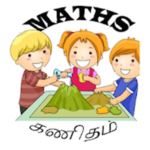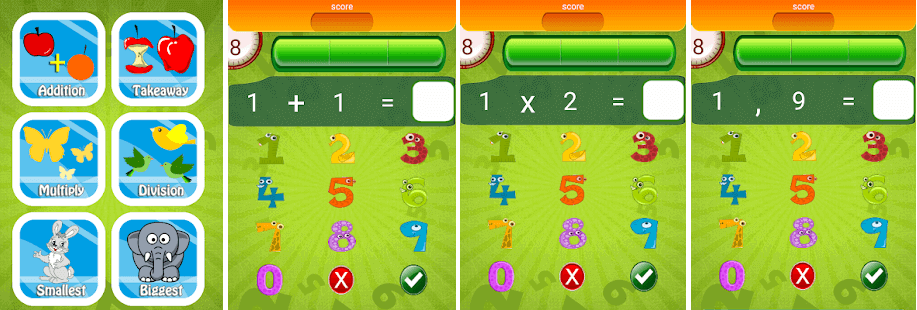# Kids Maths

## Kids MathsThe application will help kids to learn maths easily.

This is a good brain test and you can improve your maths calculations speed and It is a new educational app that focuses on numbers and maths learning. This colourful game presents a collection of maths exercises for kids from 6 to 16 years, divided into several categories based on difficulty.

The app includes the following topics: addition, subtraction, multiplication, division, number sorting, counting and comparison. This way, children can exercise various areas and not get bored of doing the same tasks over and over again.

• Learn how to calculate number with:
•  Addition game (Easy, Normal, Hard)
• Subtraction game (Easy, Normal, Hard)
•  Multiplication game (Easy, Normal, Hard)
•  Division game (Easy, Normal, Hard)